Home MonkeyNotes Printable Notes Digital Library Study Guides Study Smart Parents Tips College Planning Test Prep Fun Zone Help / FAQ How to Cite New Title Request

 (2)   If   f(x) £ on [ a,b ] then the area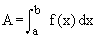i.e. the area lying above the graph of y = f (x), below the x-axis and between the ordinates x = a and x = b is       i.e.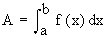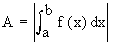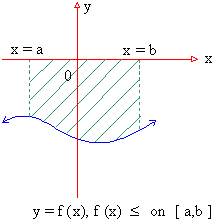(3) If F (X)³ 0 ON [ a, c ] and f (x) £ 0 on [ c, b ], then the area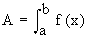of the region bounded by y = f (x), the x-axis and the ordinates x = a and x = b, is determined by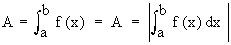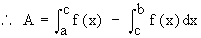So it is clear that one has to determine all points at which the graph of y = f (x) crosses the x-axis and the sign of  f  on each interval. Remark : Inter changing x and y, it follows that the area bounded by a continuous curve x = g (y), the y-axis and the lines y = a and y = b is given by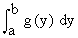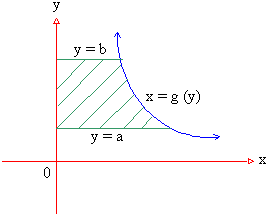Index Chapter 1